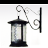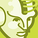# QlikView App Dev

Discussion Board for collaboration related to QlikView App Development.

Announcements
QlikWorld 2023, a live, in-person thrill ride. April 17 - 20, 2023, in Las Vegas! REGISTER TODAY
cancel
Showing results for
Did you mean:Not applicable

## Price - Volume - Mix effect analysis? (PVM)

Been trying to create a PVM-analysis without success.

Price Volume Mix effect

13 RepliesContributor III

Can I also have the template that you used?  Is this done in Pivot Table?Master II

Hi,

basically we break it into 4 parameters:

Δ PY: Amount YTD - Amount PYTD      // pretty easy, it is the simple difference

Δ Curr: Amount YTD - Amount PYTD (calculated at actual rate of exchange)     // currency-effect

Δ V: (Volume YTD - Volume PYTD)  *  Price PY (calculated at actual rate of exchange // Volume-Effect

Δ P: Δ PY - Δ Curr - Δ V     // Price-Effect

The tricky part is to calculate the Price and to define the level on which to calculate. Here you will need to use the AGGR-function, below is the function used here:

....

SUM(

{\$<

ResultPeriod_Mn = {"<= \$(=MAX(ResultPeriod_Mn) -12)"},

ResultPeriod_Y = {"\$(=MAX(ResultPeriod_Y) -1)"},

ResultPeriod_YM =,

ResultPeriod_M =>}

Amount_DOC_PY_actROE)

/

SUM(

{\$<

ResultPeriod_Mn = {"<= \$(=MAX(ResultPeriod_Mn) -12)"},

ResultPeriod_Y = {"\$(=MAX(ResultPeriod_Y) -1)"},

ResultPeriod_YM =,

ResultPeriod_M =

>}

Quantity_CON_CY))

,
ResultPeriod_M, CostGroup, SubArea)

....

In this example the cost at current exchange-rates is calculated in the script (Amount_DOC_PY_actROE).

The price is calculated on the level of the month (ResultPeriod_M), and two more groupings.

HTH

PeterContributor

Hi,

Have anyone tried to do this analysis for restaurant?

I mean, not simply analysing Price and Volume (with Mix effect, of course), but including such components as guests (not equal to volume), average bill, dishes on 1 guest e.t.c.Creator

This seems to be a very old thread, but even so here is my view.  PVM analysis isn't really much more than a series of reasonably basic mathematical equations, but some of them aren't necessarily easy to follow because the concepts, especially "Mix" which combines some concepts together and can feel a bit sticky to walk through.

There is a lot of things online, but I have found this YT tutorial the most easy to understand of all the ones I watched recently:

https://youtu.be/boMhDeQGWts

It's clear and well paced, and the way the equations work is well explained.  Others I have watched talk too fast and they over complicate their equations.  I have observed that different authors construct their equations in slightly different ways, but they reach the same answer.

If you have your data already, then all you need to do it slowly build the equations using the guide.  I thoroughly recommend if you employ the autocalendar within your Qlik Sense Load Script it makes the delimitation of periods much easier as you are readily able to access things like [date.autocalendar.YearsAgo] etc.

The video above focuses on Price, Volume and Mix only.  But I strongly recommend also building in "new" and "discontinued" data outcomes into your data solution  because trying to correctly interpret the results using just Price, Volume and Mix can be very difficult when you have "new" and "discontinued" outcomes that should be considered in isolation.

I found working the formulas using a mix of variables and master measures the easiest.

My overall advice is start in Excel, grasp the concepts, then work in Qlik Sense.Community Browser